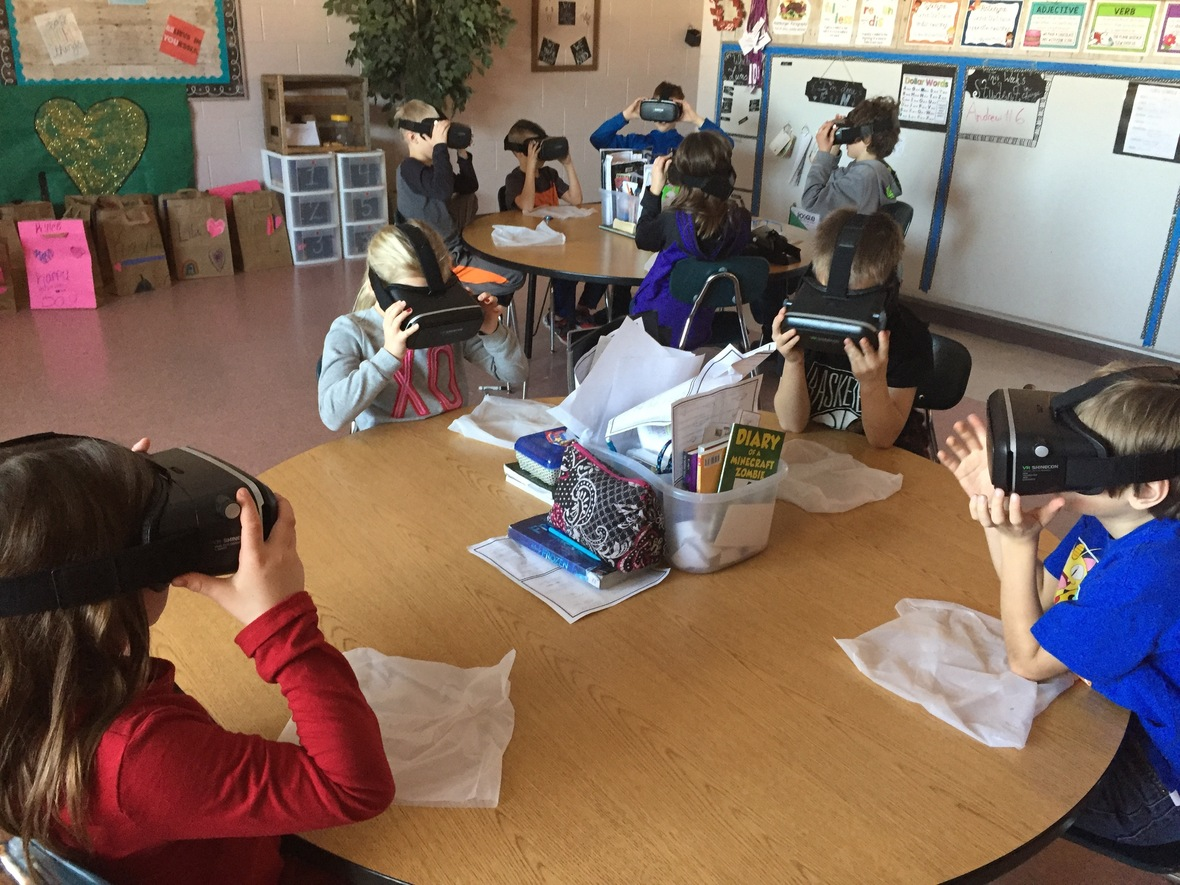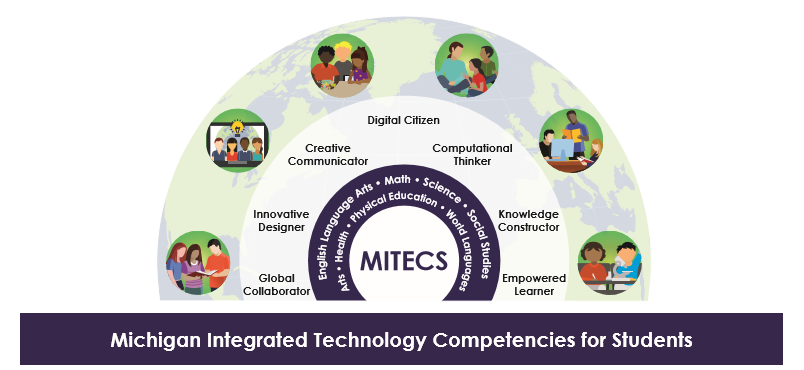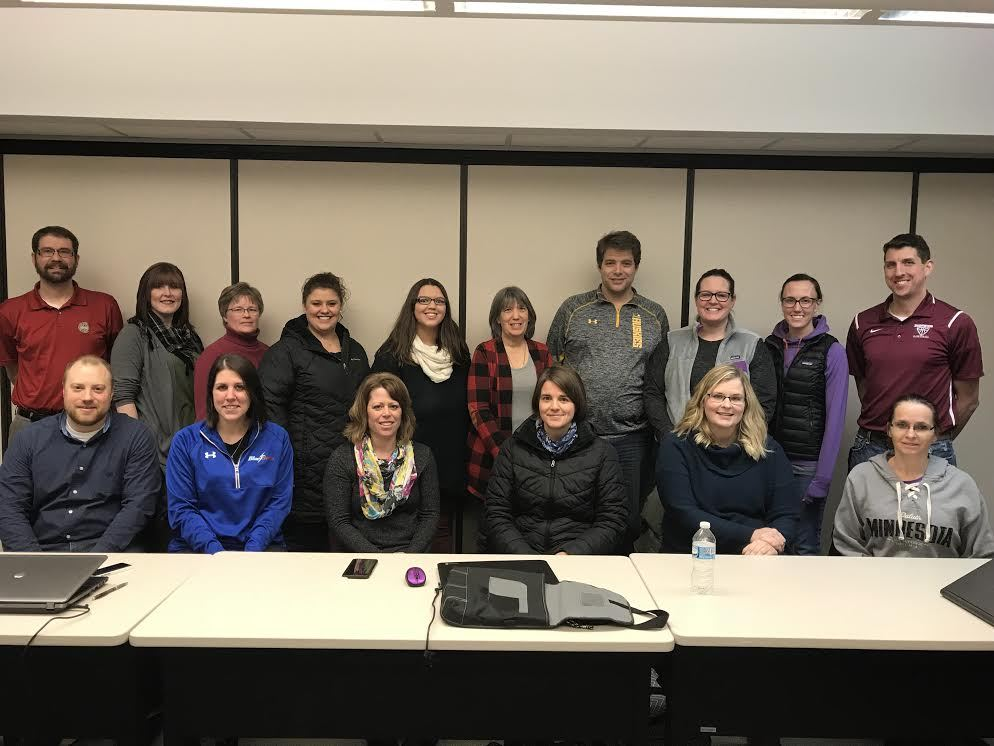Like   Tweet   +1
 /* styles */

## March 2018

 table div table+table+table+table div table{width:100%;padding:0}table div table+table+table+table div table img{width:96.23%;padding:0;float:none}table div table+table+table+table div table td{width:100%;padding:0 1.88% 18px}/* styles */## Virtual Reality KitsSouth Range Elementary had a chance to utilize one of the REMC1 Virtual Reality Kits. According to 3rd Grade Teacher, Nikki Lishinski, "The VR kit has been a huge hit!!! The kids loved the Google Expeditions tour of the Revolutionary War, and the Olympic app was a huge hit!!"

REMC1 Educational Technologist, Steve Kass, held a Virtual Reality in the Classroom workshop at the CCISD on March 1st. He will be holding this workshop again March 29th at GOISD and April 24th at CCISD. Registration is available on Wisdomwhere.
Wisdomwhere CCISD
Wisdomwhere GOISD
(For GOISD registration, peer to CCISD to find and register)

 table div table+table+table+table+table+table+table div table{width:100%;padding:0}table div table+table+table+table+table+table+table div table img{width:96.23%;padding:0;float:none}table div table+table+table+table+table+table+table div table td{width:100%;padding:0 1.88% 18px}/* styles */## New Michigan Technology CompetenciesThe Michigan Integrated Technology Competencies for Students (MITECS), released December 2017, replace the 2009 Michigan Educational Technology Standards for Students (METS-S). These updated competencies focus on learning enhanced by technology, rather than on technology tools. The MITECS are aligned to the Top 10 in 10 focus area of Learner-Centered Supports, reflecting a learner-driven approach that empowers students to be active participants in determining their educational pursuits.

The MITECS support learning in all content areas.

 table div table+table+table+table+table+table+table+table+table+table div table{width:100%;padding:0}table div table+table+table+table+table+table+table+table+table+table div table img{width:96.23%;padding:0;float:none}table div table+table+table+table+table+table+table+table+table+table div table td{width:100%;padding:0 1.88% 18px}/* styles */Bottom Row (left to right): Steve Kass, Kim Peltier, Kathy Makela, Amanda Hermanson, Jessica Klein, and Dawn Vanlerberghe Top Row (left to right): Bob Genisot, Autumn Lake, Melanie Harmala, Nikki Lishinski, Hilary Sundblad, Leona Blessing, Darin Goke, Emily Maxson, Jennifer Rubin and Tom Mott

Teachers from the schools of the Copper Country and Gogebic-Ontonagon Intermediate School Districts traveled to Bergland for the Winter Tech Academy on Friday, February 16. The group connected to the Michigan Educational Technologist Winter Virtual Rally to attend a presentation offered by Dr. Liz Kolb from the University of Michigan. Dr. Kolb talked about ways of achieving authentic engagement through technology. After the Winter Virtual Rally, the Tech Academy got the opportunity to experience a way of incorporating authentic engagement through the use of Google Expeditions and the new virtual reality kit!

 table div table+table+table+table+table+table+table+table+table+table+table+table+table div table{width:100%;padding:0}table div table+table+table+table+table+table+table+table+table+table+table+table+table div table img{width:96.23%;padding:0;float:none}table div table+table+table+table+table+table+table+table+table+table+table+table+table div table td{width:100%;padding:0 1.88% 18px}/* styles */## Meet Our Staff!We have a new employee that has joined our REMC1 helpdesk team, David Skotarcyzk.
David moved to the Copper Country from Detroit in 2004. He has a degree in Graphic Design from Westwood College. In addition to working at REMC, David owns a company that offers computer sales and service, TV repair, and graphic and web design. Welcome David!

 table div table+table+table+table+table+table+table+table+table+table+table+table+table+table+table+table div table{width:100%;padding:0}table div table+table+table+table+table+table+table+table+table+table+table+table+table+table+table+table div table img{width:96.23%;padding:0;float:none}table div table+table+table+table+table+table+table+table+table+table+table+table+table+table+table+table div table td{width:100%;padding:0 1.88% 18px}/* styles */## Teacher's CornerA recent report from Discovery Education links multiple studies linking streaming video usage to increased student engagement and achievement.

 table div table+table+table+table+table+table+table+table+table+table+table+table+table+table+table+table+table+table+table div table{width:100%;padding:0}table div table+table+table+table+table+table+table+table+table+table+table+table+table+table+table+table+table+table+table div table img{width:96.23%;padding:0;float:none}table div table+table+table+table+table+table+table+table+table+table+table+table+table+table+table+table+table+table+table div table td{width:100%;padding:0 1.88% 18px}/* styles */## ResourcesEduPaths is a free resource from TRIG created to centralize PD around the state. Find resources and get FREE SCECH hours!

Current REMC Connect (REMC Association of Michigan Newsletter)

Check out Discovery Streaming updates and enhancing instruction with streaming resources.

MeL is a great place for Michigan Teachers to find classroom resources. They have just recently launched MeL Teachers which offers eight centers that will help with site navigation and make important information easier to access.

REMC Virtual Courses, April, 2018. Free to Michigan school personnel. Sessions include: Open Education Resources, Google Apps for Chrome, Literacy and Technology, Social Media and more. SCECHs available. FAQs about the free REMC Virtual Courses

 table div table+table+table+table+table+table+table+table+table+table+table+table+table+table+table+table+table+table+table+table+table+table div table{width:100%;padding:0}table div table+table+table+table+table+table+table+table+table+table+table+table+table+table+table+table+table+table+table+table+table+table div table img{width:96.23%;padding:0;float:none}table div table+table+table+table+table+table+table+table+table+table+table+table+table+table+table+table+table+table+table+table+table+table div table td{width:100%;padding:0 1.88% 18px}/* styles */## Connection CornerDiscovery Education upcoming events
Video Conference field trips and Classroom collaborations
iNACOL webinars
ISTE blog and magazine
EdTech magazine
REMC SAVE
Michigan Ed Tech - METS

 table div table+table+table+table+table+table+table+table+table+table+table+table+table+table+table+table+table+table+table+table+table+table+table+table+table div table{width:100%;padding:0}table div table+table+table+table+table+table+table+table+table+table+table+table+table+table+table+table+table+table+table+table+table+table+table+table+table div table img{width:96.23%;padding:0;float:none}table div table+table+table+table+table+table+table+table+table+table+table+table+table+table+table+table+table+table+table+table+table+table+table+table+table div table td{width:100%;padding:0 1.88% 18px}/* styles */table div table+table+table+table+table+table+table+table+table+table+table+table+table+table+table+table+table+table+table+table+table+table+table+table+table+table+table div table{width:100%;padding:0}table div table+table+table+table+table+table+table+table+table+table+table+table+table+table+table+table+table+table+table+table+table+table+table+table+table+table+table div table img{width:96.23%;padding:0;float:none}table div table+table+table+table+table+table+table+table+table+table+table+table+table+table+table+table+table+table+table+table+table+table+table+table+table+table+table div table td{width:100%;padding:0 1.88% 18px}/* styles */table div table+table+table+table+table+table+table+table+table+table+table+table+table+table+table+table+table+table+table+table+table+table+table+table+table+table+table+table+table div table{width:100%;padding:0}table div table+table+table+table+table+table+table+table+table+table+table+table+table+table+table+table+table+table+table+table+table+table+table+table+table+table+table+table+table div table img{width:96.23%;padding:0;float:none}table div table+table+table+table+table+table+table+table+table+table+table+table+table+table+table+table+table+table+table+table+table+table+table+table+table+table+table+table+table div table td{width:100%;padding:0 1.88% 18px}/* styles */table div table+table+table+table+table+table+table+table+table+table+table+table+table+table+table+table+table+table+table+table+table+table+table+table+table+table+table+table+table+table+table div table{width:100%;padding:0}table div table+table+table+table+table+table+table+table+table+table+table+table+table+table+table+table+table+table+table+table+table+table+table+table+table+table+table+table+table+table+table div table img{width:96.23%;padding:0;float:none}table div table+table+table+table+table+table+table+table+table+table+table+table+table+table+table+table+table+table+table+table+table+table+table+table+table+table+table+table+table+table+table div table td{width:100%;padding:0 1.88% 18px}/* styles */